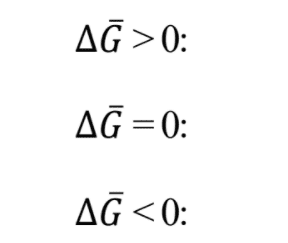# Thermodynamic meaning of molar Gibbs free energy

• GranMix

#### GranMix

Homework Statement
What's the thermodynamic meaning of these molar Gibbs free energy values?
Relevant Equations
See the values below.I know that when it is ΔG>0 , it means there is no spontaneity, when ΔG=0 there is equilibrium, and when ΔG<0, there is spontaneity. But what happens when this is in the context of partial molar properties, when G is molar?
I suppose molar ΔG is referred to a solution. Right? In that case, is it a change due to mixture? If so, what does this values mean?

Homework Statement:: What's the thermodynamic meaning of these molar Gibbs free energy values?
Relevant Equations:: See the values below.

View attachment 272847
I know that when it is ΔG>0 , it means there is no spontaneity, when ΔG=0 there is equilibrium, and when ΔG<0, there is spontaneity. But what happens when this is in the context of partial molar properties, when G is molar?
I suppose molar ΔG is referred to a solution. Right? In that case, is it a change due to mixture? If so, what does this values mean?
You seem very confused about all this. I'm having trouble figuring out where to begin.

First of all, what do you mean by those overbears above the G's?

Secondly, those ##\Delta G## should be ##\Delta G^0##? These refer to the change between two specific end states. Do you know what those two end states are for gas reaction?

Thirdly, the spontaneity relationships are only rules of thumb. All reactions are spontaneous, but the different inequalities refer to the relative tendencies for the reaction starting from reactants to go to completion.

Now, for partial molar free energy of a species in a mixture. The total free energy of a mixture is expressible as ##G=\sum{n_j \mu_j}## where the n's are the numbers of moles of the species in the mixture and the ##\mu##'s are equal to partial molar free energy (aka the chemical potential) of the species, defined as follows: $$\mu_j=\left(\frac{\partial G} {\partial n_j}\right)_{T,P,n_k}$$ For the specific situation of an ideal gas mixture, do you know the equation for the chemical potential of each individual species in terms of the species mole fraction and the molar free energy of formation of the pure species?

•GranMix
The subject of this homework is partial molar properties, so chemical reactions are not involved. The overbears above the G's mean molar, as it doesn't have a subscript, i guess it refers to the change of molar free energy of the solution, not of a specie. Also, my course doesn't includes chemical potential, so i think it doesn't have a relationship to it. So i think it has to be with explaining if there is spontaneity or equilibrium in those three values for a solution.

The subject of this homework is partial molar properties, so chemical reactions are not involved. The overbears above the G's mean molar, as it doesn't have a subscript, i guess it refers to the change of molar free energy of the solution, not of a specie. Also, my course doesn't includes chemical potential, so i think it doesn't have a relationship to it. So i think it has to be with explaining if there is spontaneity or equilibrium in those three values for a solution.
Chemical potential is the name given to partial molar Gibbs free energy. They are the same thing.

Chemical potential is the name given to partial molar Gibbs free energy. They are the same thing.
Yes. But in this case the molar ΔG given is not partial, it is the total free energy of a mixture . So i think that without considering its definition related to chemical potential (because my course doesn't include that), i must explain if there is spontaneity or equilibrium for these values. I insist in this because in class my teacher has emphasized many times the following:when ΔG>0 in a process , it means there is no spontaneity, when ΔG=0 there is equilibrium, and when ΔG<0, there is spontaneity. So it surely has to be related to this. The difference is that now the ΔG is molar and for a mixture.

Yes. But in this case the molar ΔG given is not partial, it is the total free energy of a mixture . So i think that without considering its definition related to chemical potential (because my course doesn't include that), i must explain if there is spontaneity or equilibrium for these values. I insist in this because in class my teacher has emphasized many times the following:when ΔG>0 in a process , it means there is no spontaneity, when ΔG=0 there is equilibrium, and when ΔG<0, there is spontaneity. So it surely has to be related to this. The difference is that now the ΔG is molar and for a mixture.
Like I said, the total ##\Delta G## is related to the change in G between a specific initial state and a specific final state. And I am asking you to identify what these initial and final states are. If you are learning this properly, you should be able to specify precisely what these two states are. You should also be able to tell how the ##\Delta G## between these states is related to the equilibrium constant, so that you can better understand how it is related to so-called "spontaneity."

You also need to be able start with the equation ##G=\sum{n_j \mu_j}## for an ideal gas mixture (for which the partial molar free energy of each species is expressed as ##\mu_j=\mu_j^0+RT\ln{p_j}##, with ##\mu_j^0## representing the free energy of formation and ##p_j## representing the partial pressure), and find the conversion of the reaction that minimizes G. This will lead to the equation for the equilibrium constant in terms of ##\Delta G^0##.

•GranMix